### 背景 && 摘要

1. IDA 远程调试设置
2. 使用 IDA Keypatch 插件对程序打补丁
3. 了解并能够识别 rc4 加密算法
4. setjmp/longjmp
5. 简单虚拟机指令的分析方法
6. 根据 IDA 伪代码分析程序时的细节问题

# 初步分析

```flag only appears at a specific time, range [2018-05-19 09:00, 2018-05-21 09:00)
Better luck next time :)
```

```➜  workshop file magic.exe
magic.exe: PE32+ executable (console) x86-64 (stripped to external PDB), for MS Windows
```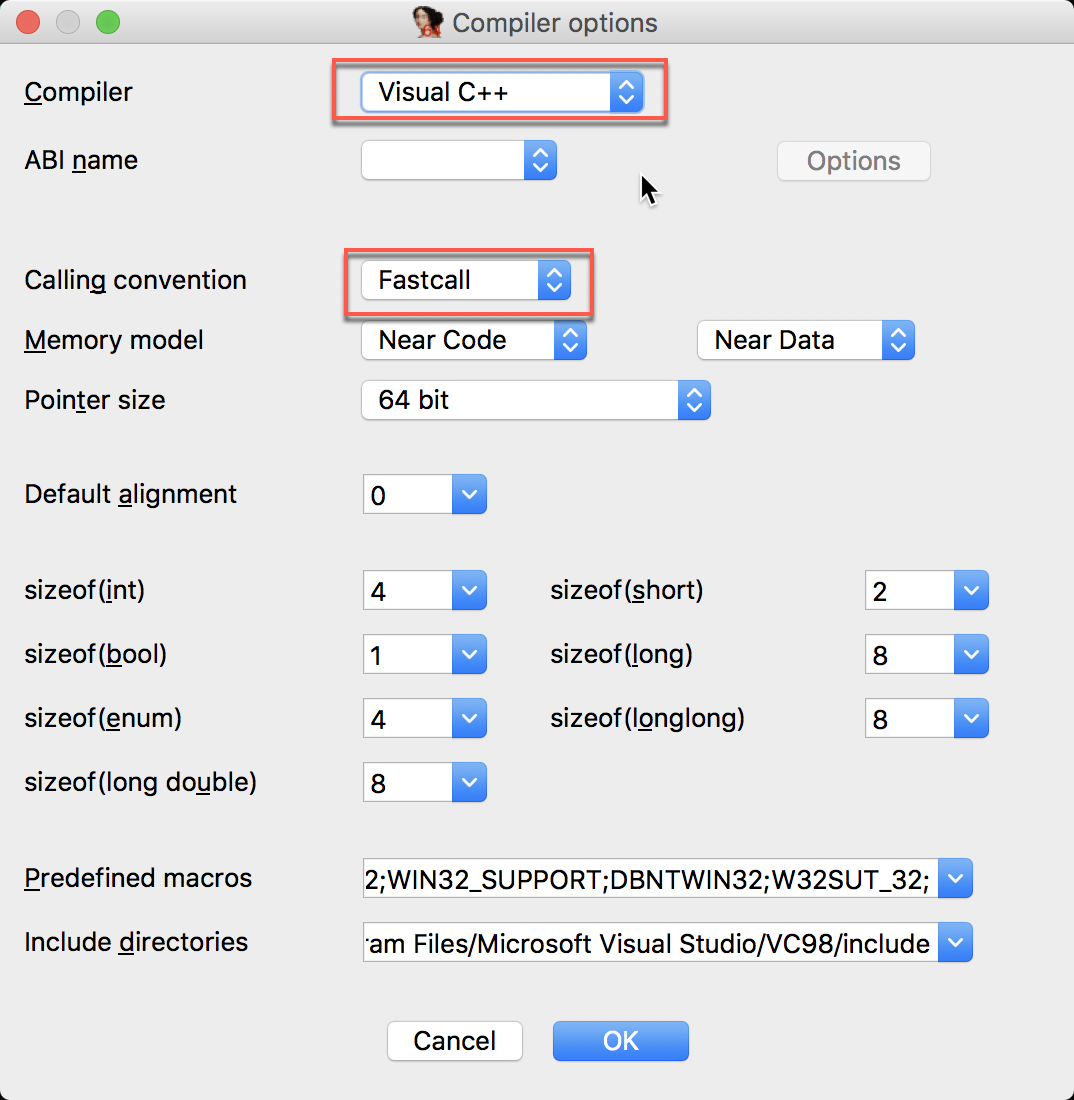```.rdata:0000000000406018	0000006A	C	flag only appears at a specific time, range [2018-05-19 09:00, 2018-05-21 09:00)\nBetter luck next time :)
```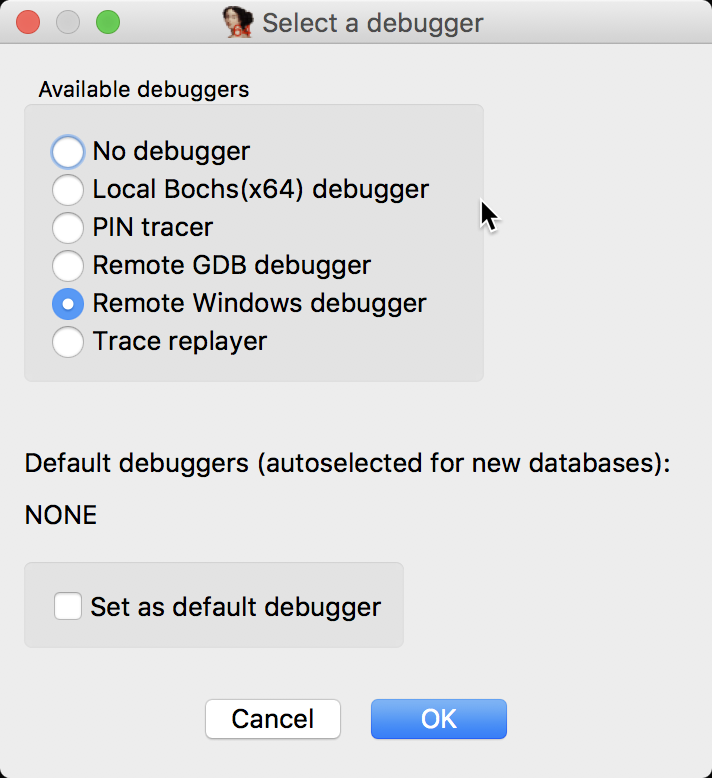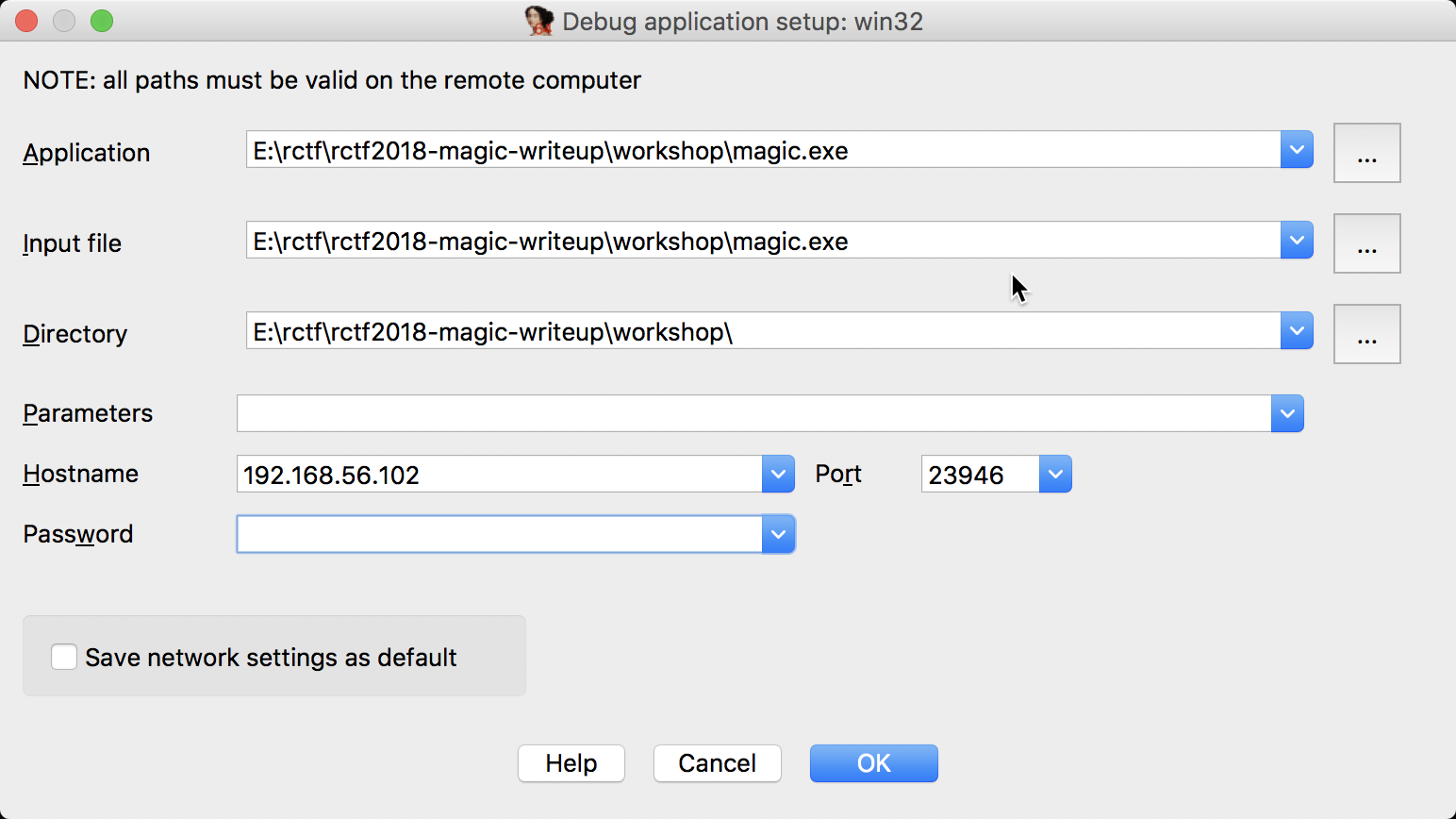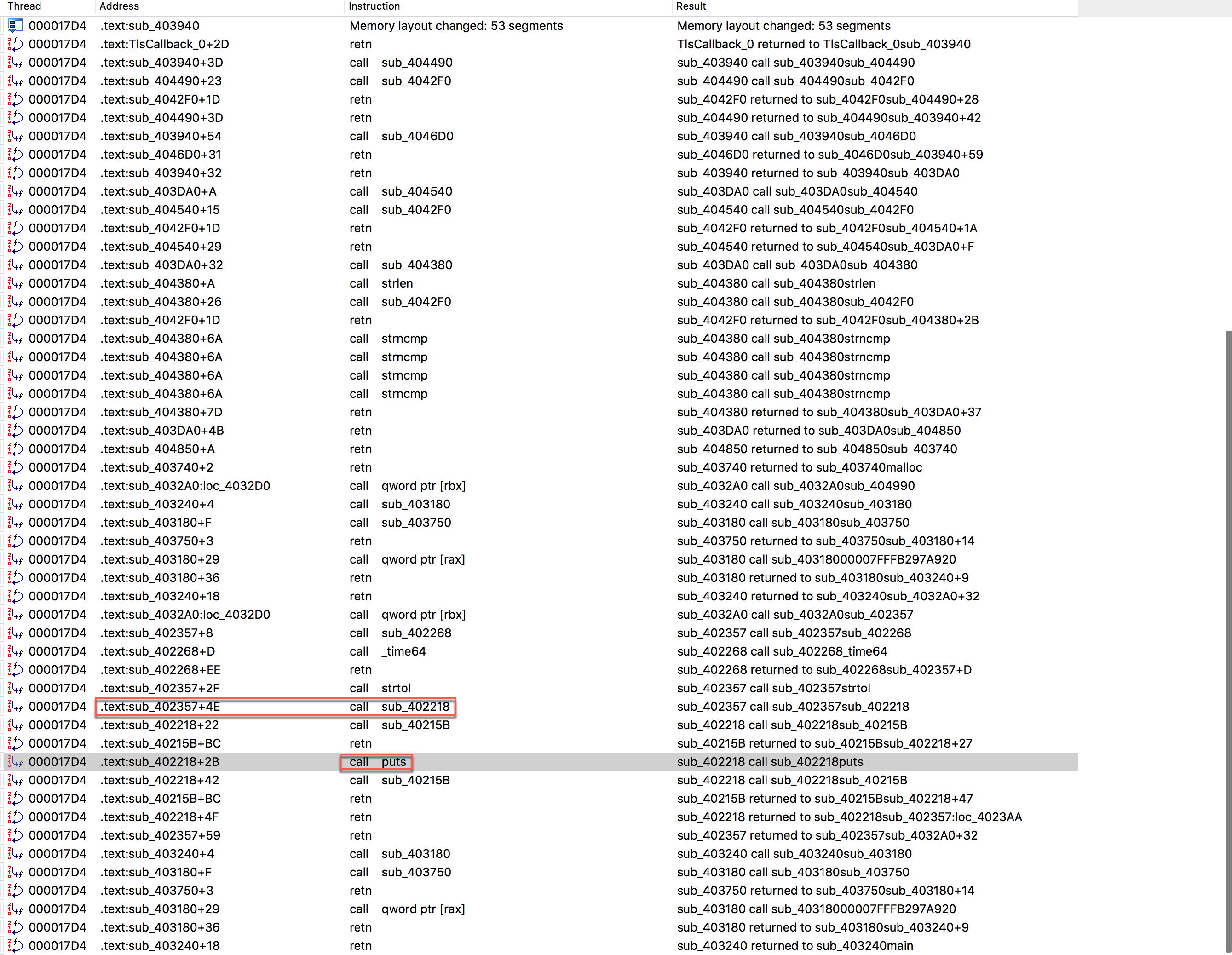sub_402218() 如下所示

```__int64 __fastcall sub_402218(const char *a1, __int64 a2, int a3, __int64 a4)
{
v7 = a1;
v8 = a2;
v9 = a3;
sub_40215B(a1, a2, a3, a4);
v4 = puts(v7);
sub_40215B(v7, v8, v9, v5);
return v4;
}
```

```__int64 __fastcall sub_402357(const char *a1, char **a2)
{
__int64 result; // rax
int v3; // eax
__int64 v4; // r9

sub_402268(a1, a2);
result = dword_4099D0;
if ( !dword_4099D0 )
{
v3 = strtol("ca11ab1e", 0i64, 16);
result = sub_402218(&unk_405220, 105i64, v3 ^ 0xBADD1917, v4);
}
return result;
}
```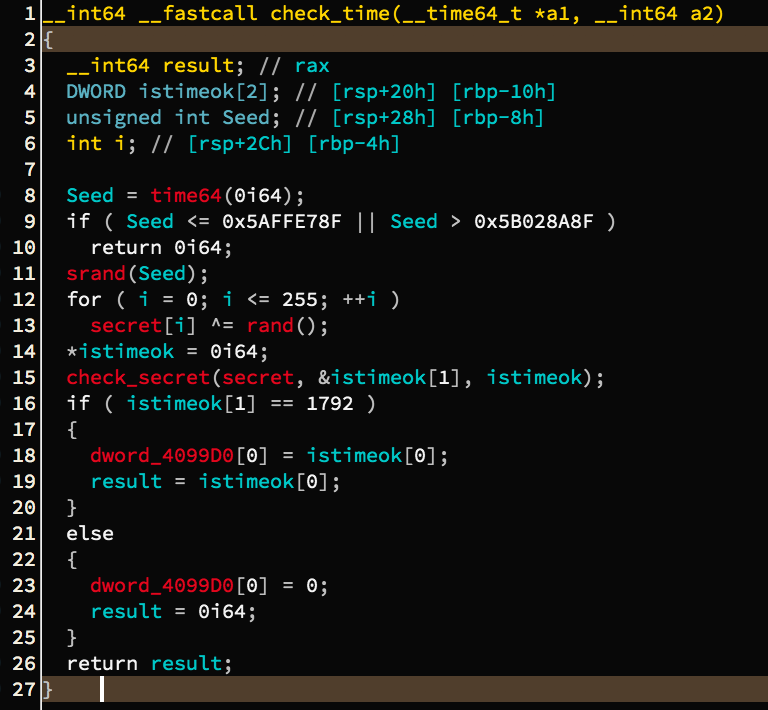# 第一阶段: 寻找正确的时间戳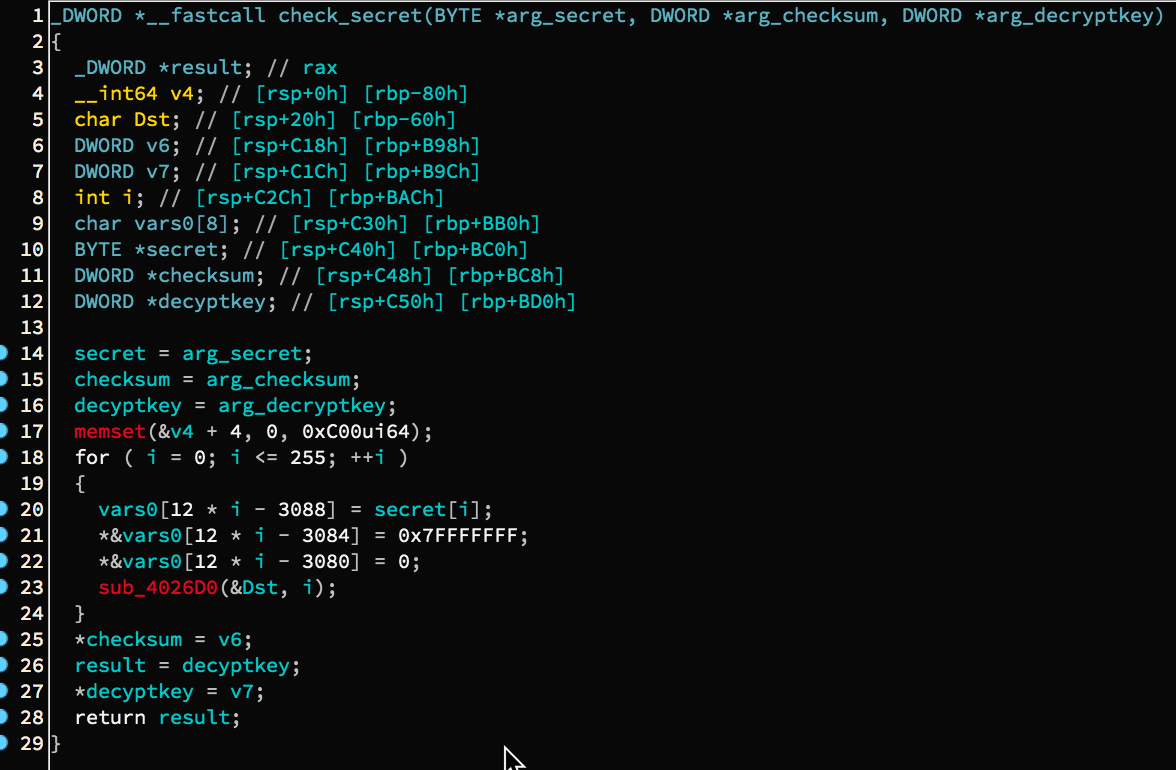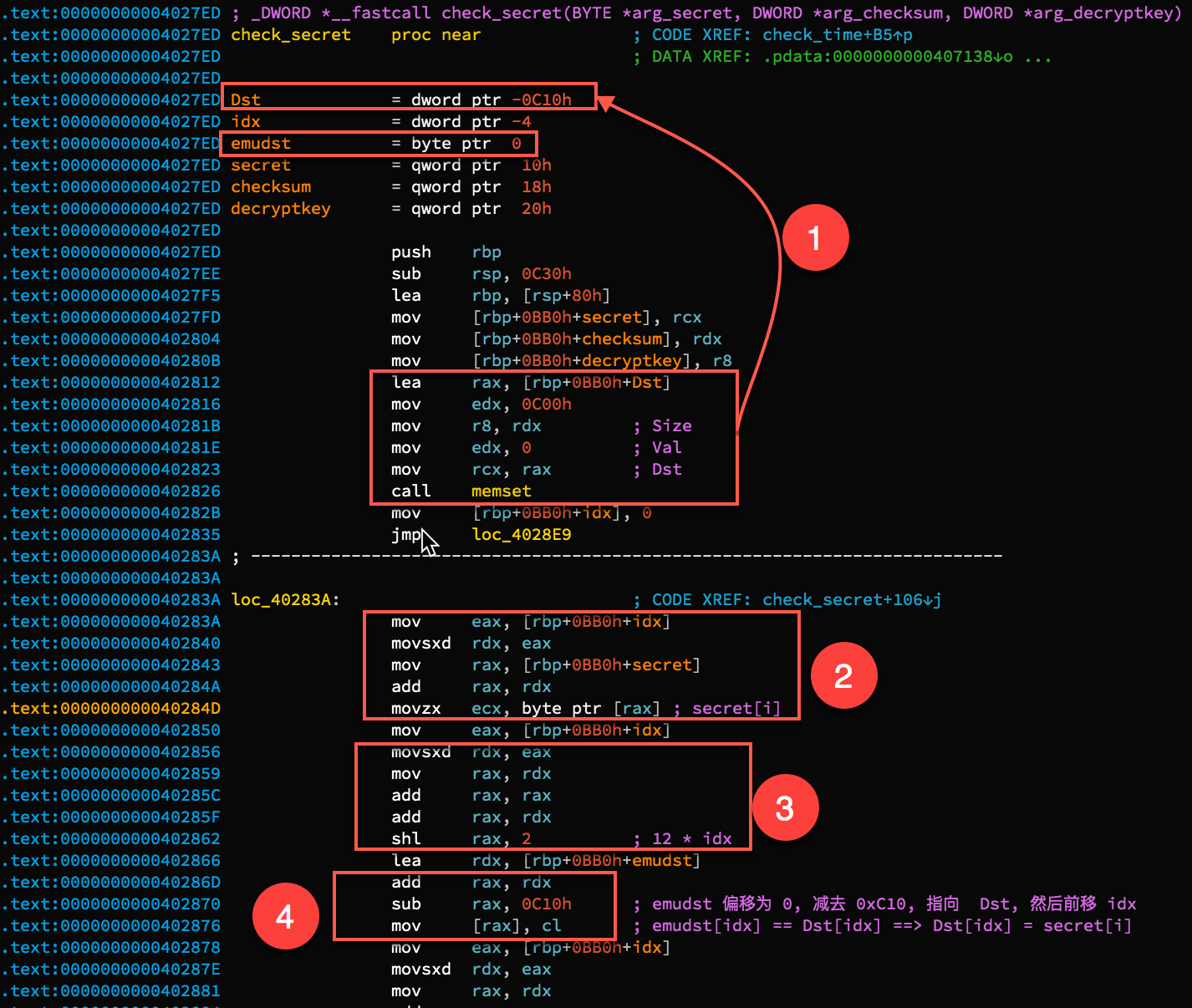1 处使用 memset 对 Dst 进行置零操作, 注意 Dst 的偏移是 -0C10h, emudst 的偏移地址是 0. 2 处获取到 secret[i], 3 处计算了一个偏移值, 每次循环向前移动 12 字节, 所以是 12 * idx, idx 表示当前索引值. 4 处是重点, 由于 emudst 偏移为 0, 减去 0C10 后, 正好指向 Dst, 然后前移 idx, 相当于 Dst[i]. 所以我们明白, 实际上, emudst 和 Dst 是同一个东西. 而且我们看到循环中每次前移 12 字节, 分为三次赋值, 所以 Dst 的类型 为 DWORD, 大小为 0xc00/ 4 = 768. 在反编译窗口中对 Dst 类型重定义为 DWORD Dst, 发现反编译代码变成如下所示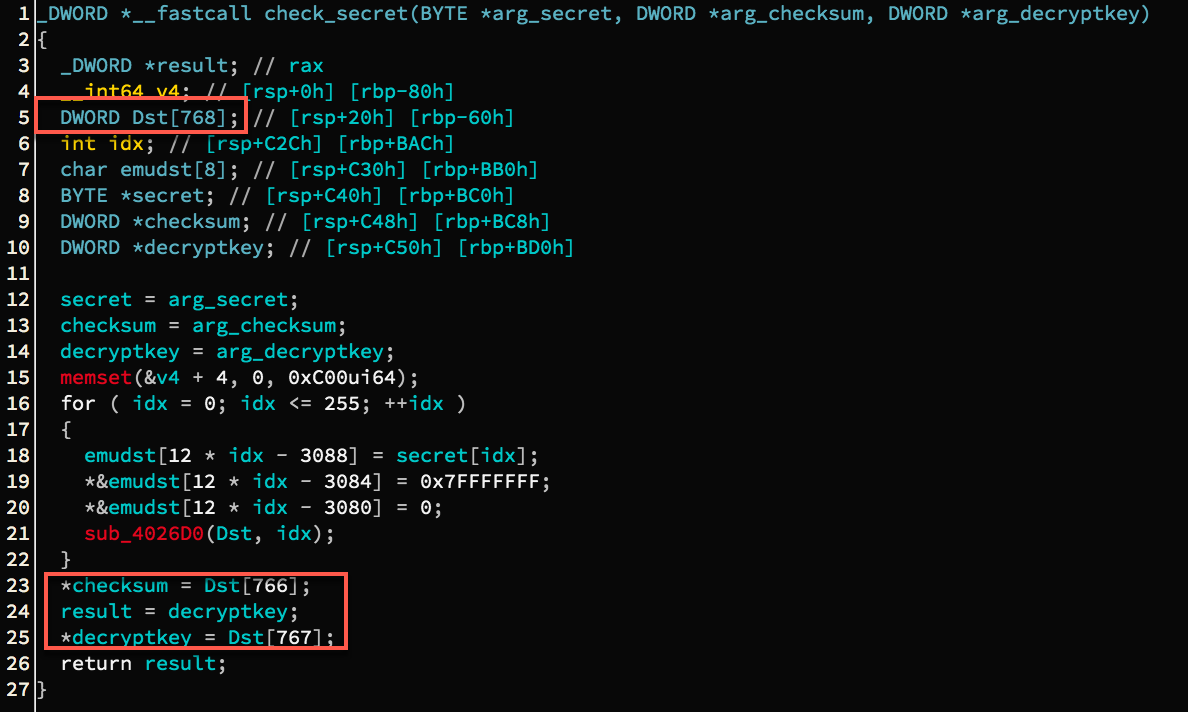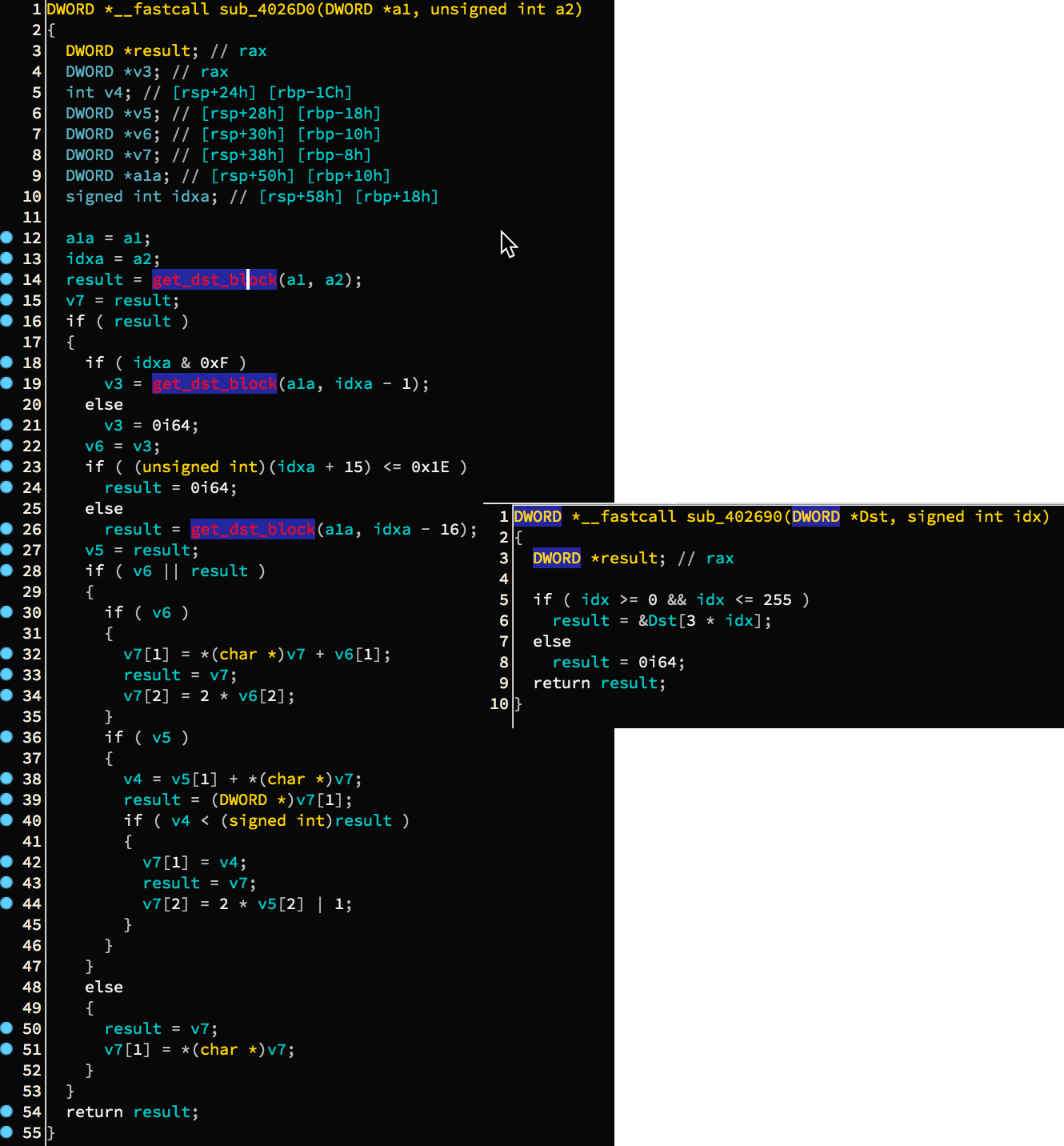```
#include <stdlib.h>
#include <time.h>
#include <stdio.h>
#include <ctype.h>
#include <string.h>
#include <inttypes.h>
#include <windows.h>

#define BYTE  uint8_t
#define WORD  uint16_t
#define DWORD uint32_t
#define QWORD uint64_t

DWORD* get_dst_block(DWORD *Dst, signed int idx)
{
DWORD *result; // rax

if ( idx >= 0 && idx <= 255 )
result = &Dst[3 * idx];
else
result = 0;
return result;
}

void sub_4026D0(DWORD *a1, unsigned int a2)
{
DWORD *result; // rax
DWORD *v3; // rax
int v4; // [rsp+24h] [rbp-1Ch]
DWORD *v5; // [rsp+28h] [rbp-18h]
DWORD *v6; // [rsp+30h] [rbp-10h]
DWORD *v7; // [rsp+38h] [rbp-8h]
DWORD *a1a; // [rsp+50h] [rbp+10h]
signed int idxa; // [rsp+58h] [rbp+18h]

a1a = a1;
idxa = a2;
result = get_dst_block(a1, a2);
v7 = result;
if ( result )
{
if ( idxa & 0xF )
v3 = get_dst_block(a1a, idxa - 1);
else
v3 = 0;
v6 = v3;
if ( (unsigned int)(idxa + 15) <= 0x1E )
result = 0;
else
result = get_dst_block(a1a, idxa - 16);
v5 = result;
if ( v6 || result )
{
if ( v6 )
{
v7 = *(char *)v7 + v6;
result = v7;
v7 = 2 * v6;
}
if ( v5 )
{
v4 = v5 + *(char *)v7;
result = (DWORD *)v7;
if ( v4 < (signed int)result )
{
v7 = v4;
result = v7;
v7 = 2 * v5 | 1;
}
}
}
else
{
result = v7;
v7 = *(char *)v7;
}
}
}

int main()
{
int min_epoch = 1526720399 + 1;
int max_epoch = 1526893199;
DWORD Dst;
BYTE buf;
for(int epoch = min_epoch; epoch <= max_epoch; ++epoch)
{
srand(epoch);
/*
* Do not write like this(since we are doing force brute....):
* for(int i = 0; i <= 255; ++i) secret[i] ^= rand();
* This is the right way:
* for(int i = 0; i <= 255; ++i) buf[i] = secret[i] ^ rand();
*/
for(int i = 0; i <= 255; ++i) buf[i] = secret[i] ^ rand();
memset(Dst,0,0xc00);
for(int i =0; i <= 255; ++i)
{
/*
* Note that this is a byte assignment, not a DWORD.
* We could confirm through the corresponding assembly
* code: "mov [rax], cl". In fact, here is an alignment
* in 4 bytes.
*/
Dst[3 * i] = buf[i];
Dst[3 * i + 1] =  0x7FFFFFFF;
Dst[3 * i + 2] = 0;
sub_4026D0(Dst, i);
}
DWORD checksum = Dst;
DWORD decryptkey = Dst;
if(checksum == 0x700)
{
printf("epoch is found!\n");
printf("EPOCH = %#x decryptkey = %#x\n", epoch, decryptkey);
break;
}
}
printf("DONE!\n");
/*
* epoch is found!
* EPOCH = 0x5b00e398 decryptkey = 0x322ce7a4
* DONE!
*/
return 0;
}

```

IDA 在强制类型转换的时候, 自己定义了一些宏指令, 这些宏指令具体意思可以在 这里 查找.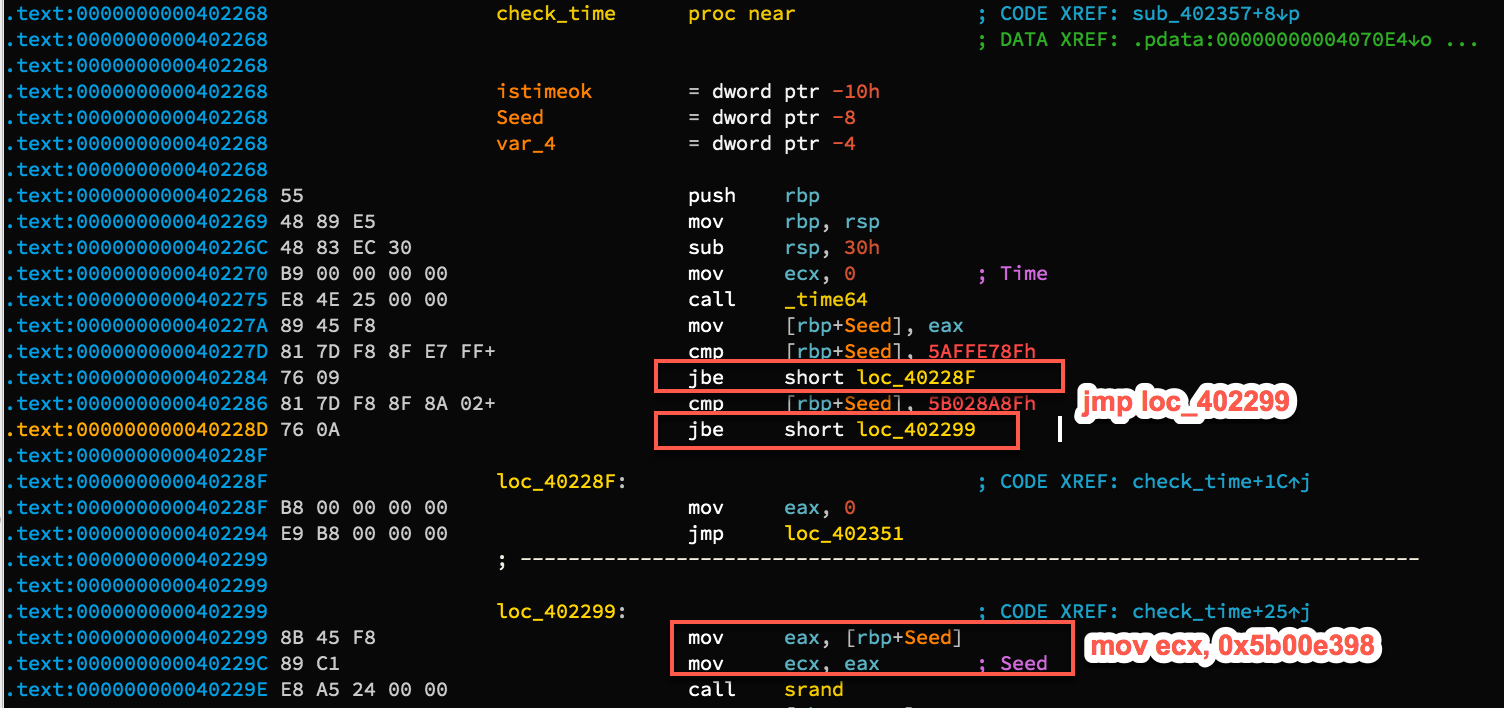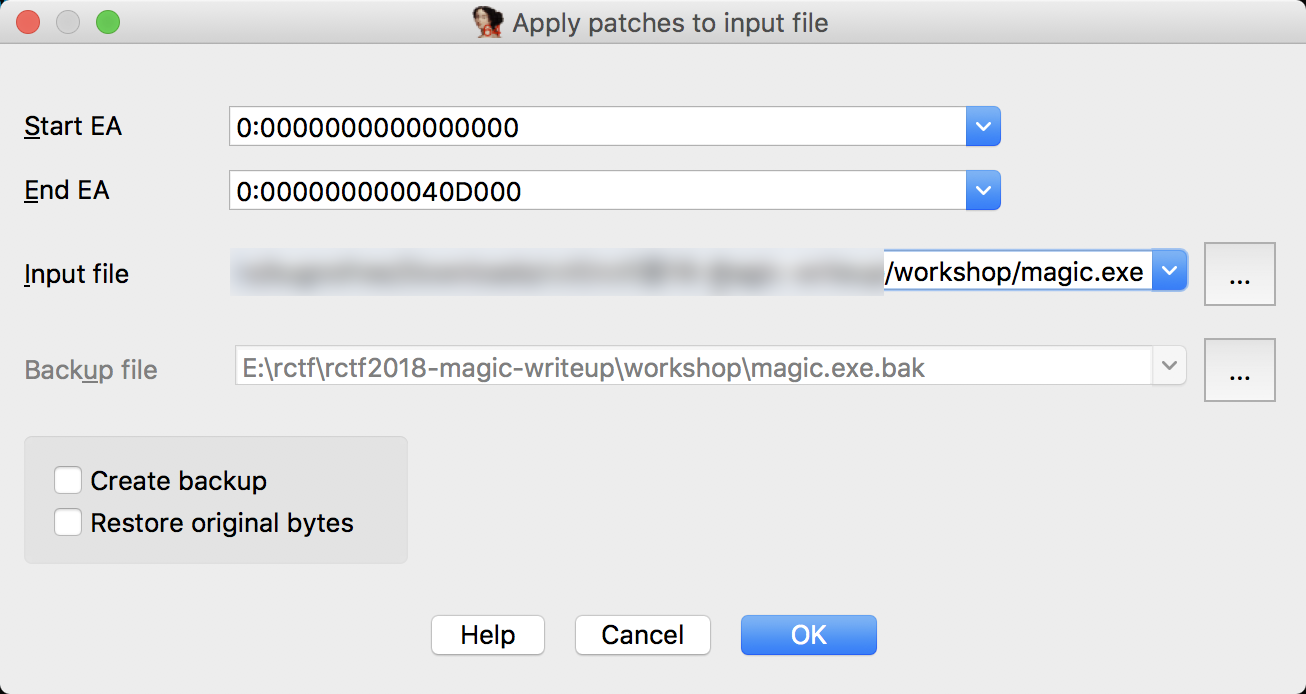# 第二阶段: RC4 算法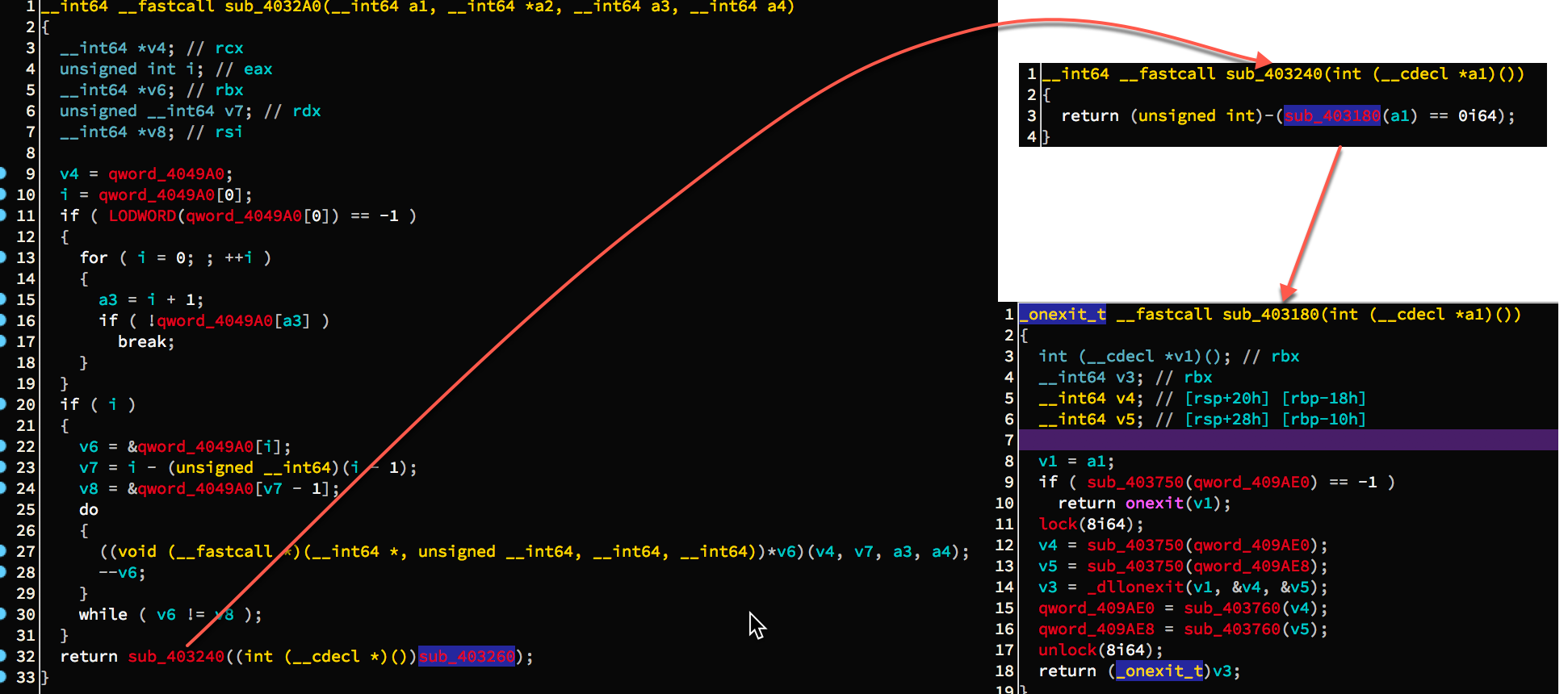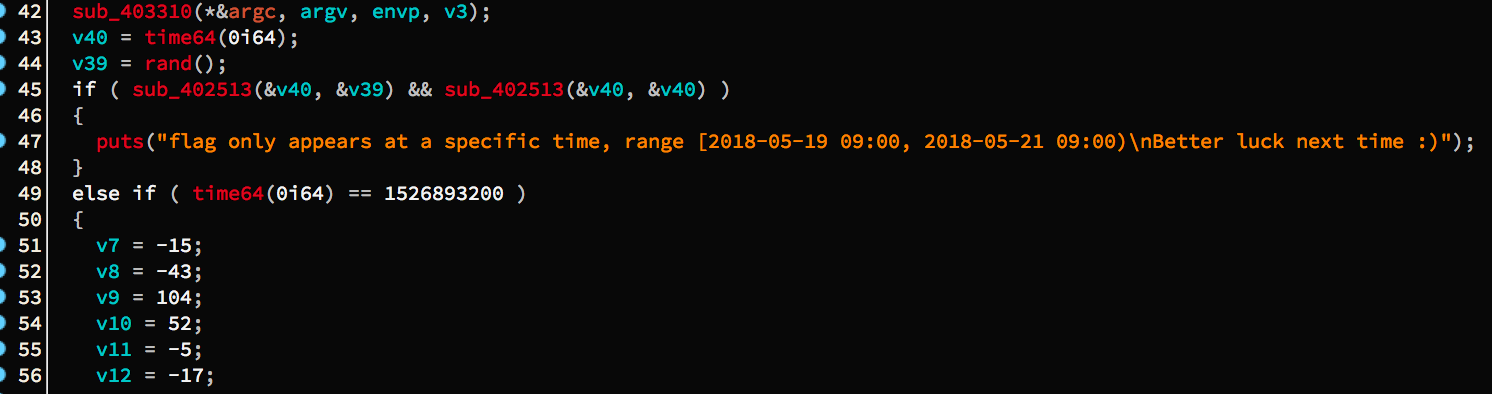sub_403310 函数进去后, 会检测自己是不是第一次执行, 如果是的话, 自己就退出. main 函数执行前, sub_403310 已经执行过一次了, 用交叉引用查看一下 main 的调用体, 然后上面就是 sub_403310. 好了, 总之到现在这个不是关键啦, 我们就不管他了.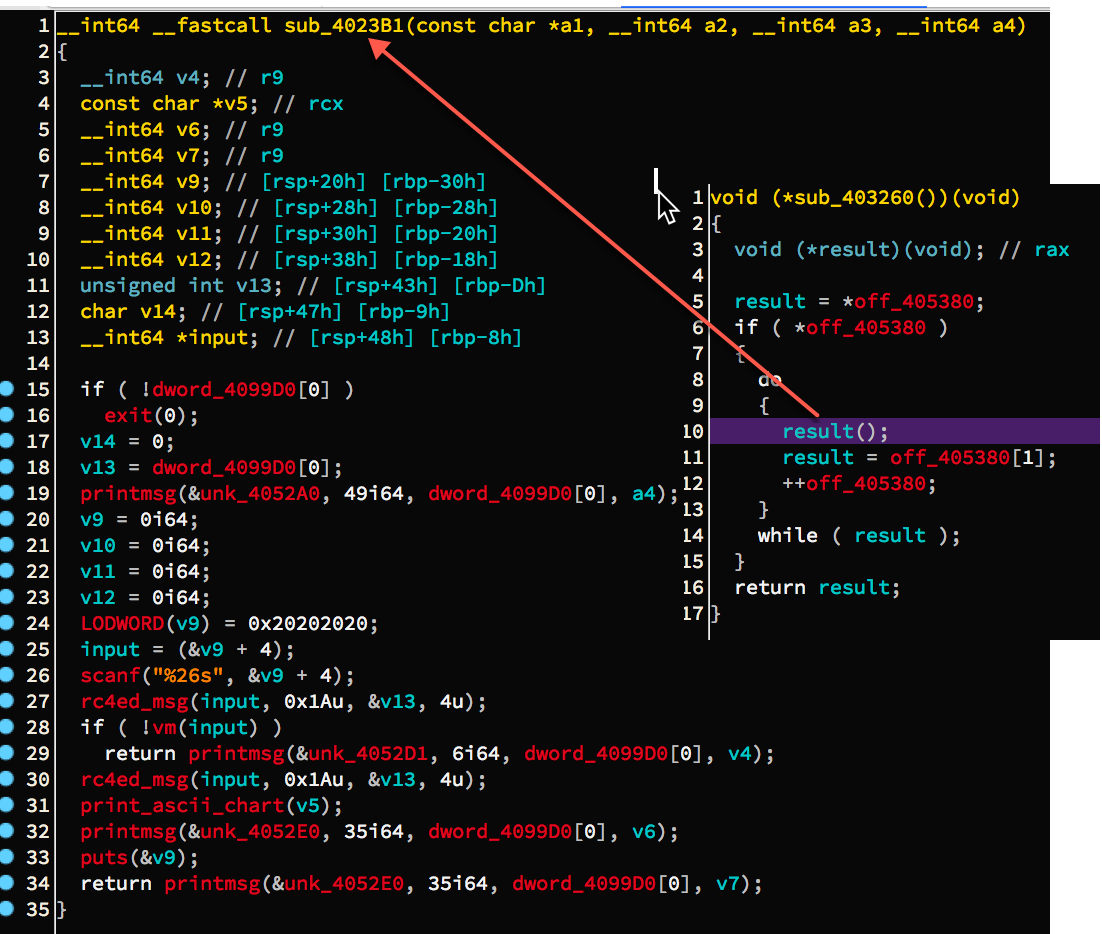rc4 加密算法分为两个阶段

• Key-scheduling algorithm (KSA, Key 调度算法)
```for i in range(256): S[i] = i
j = 0
for i in range(256):
j = (j + S[i] + key[ i % keylength ]) % 256
swap(S[i], S[j])
```
这一阶段主要用于初始化数组 S, 首先 S[i] 初始化为 i, 再对每一个 i 值, 计算下一个索引 j, 然后交换 S[i] 与 S[j].
• Pseudo-random generation algorithm (PRGA,伪随机数生成算法)
```i = 0
j = 0
while GeneratingOutput:
i = (i + 1) % 256
j = (j + S[i]) % 256
swap(S[i], S[j])
K = inputByte ^ S[(S[i] + S[j]) % 256]
output K
```
通过两个 S 盒指针, 不断交换指针处的的元素值, 再以元素值之和作为索引, 取 S 盒的元素, 最后与输入的字节流中的当前字节做异或操作, 产生一个输出.

wiki 上的一张图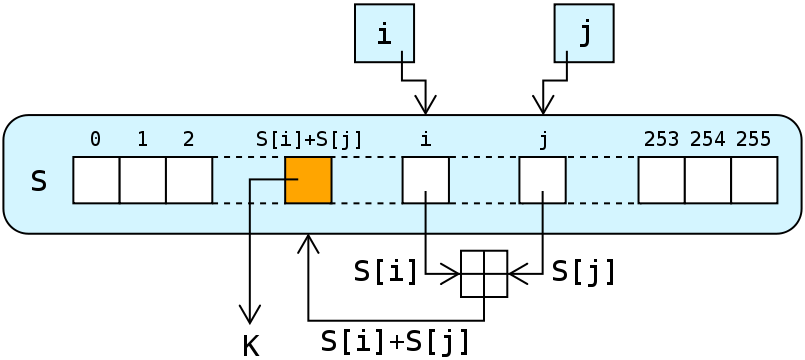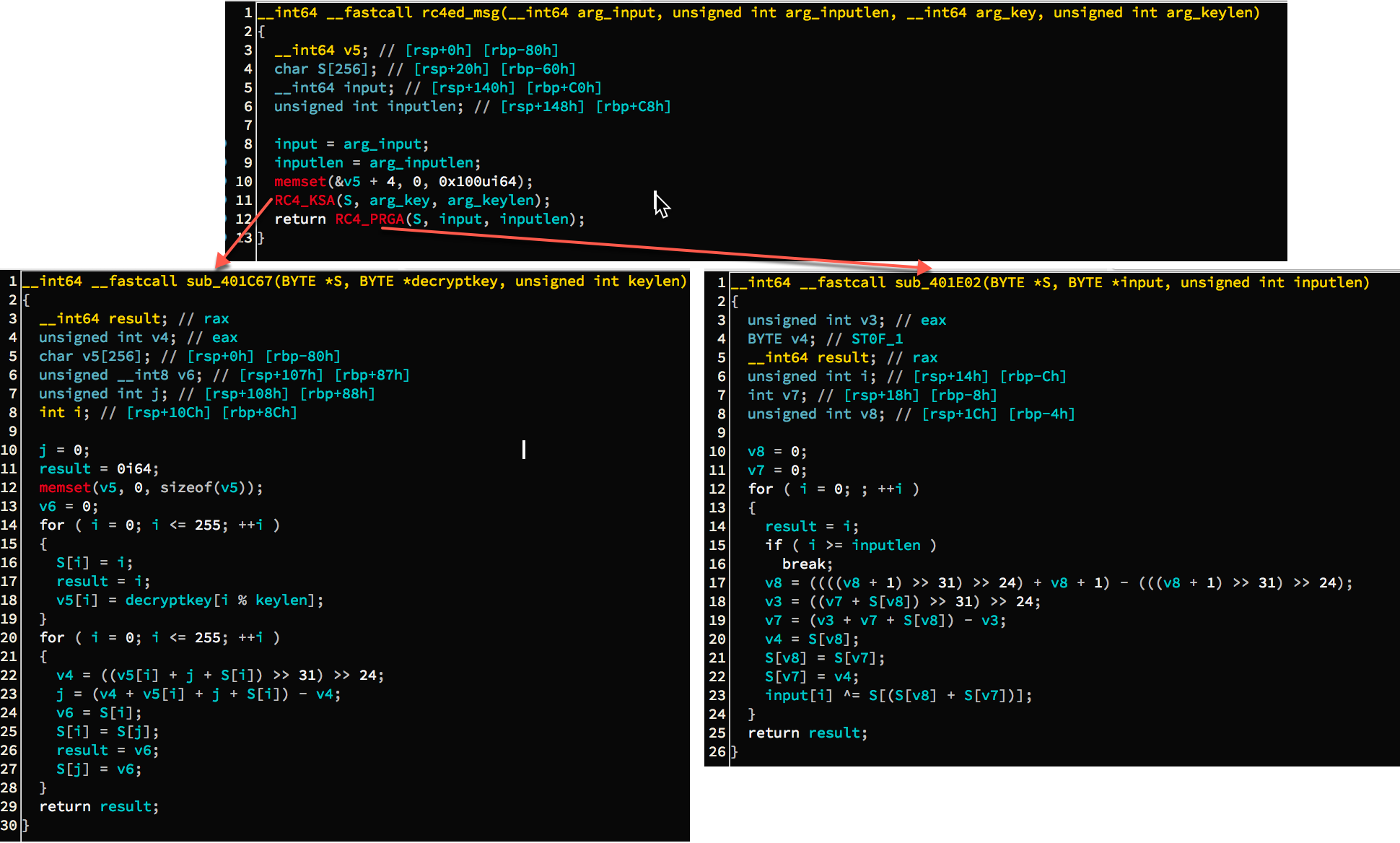KSA 阶段它的实现一模一样, 只不过多了一个 v5[i] 暂存中间变量, v4 是多余的. PRGA 阶段 v8 那一大堆实际上就是 v8 = v8 + 1, v3 是多余的. v8 和 v7 分别是两个指向 S 盒的指针. 实现都是一样的.

# 第三阶段: 虚拟机指令

```#include <setjmp.h>
int setjmp(jmp_buf env);
void longjmp(jmp_buf env, int val);
```

setjmp 保存将当前环境信息, 如寄存器的值, 保存到 env 中, longjmp 将会使用该 env 进行跳转, setjmp 执行后返回的值总是 0. longjmp 有两个参数, 第一个就是刚刚说的 env. 当 longjmp 执行完毕后, 效果就像是从 setjmp 返回, 返回值就是 longjmp 的第二个参数 val. 其执行流可以参考下图, 清晰明了.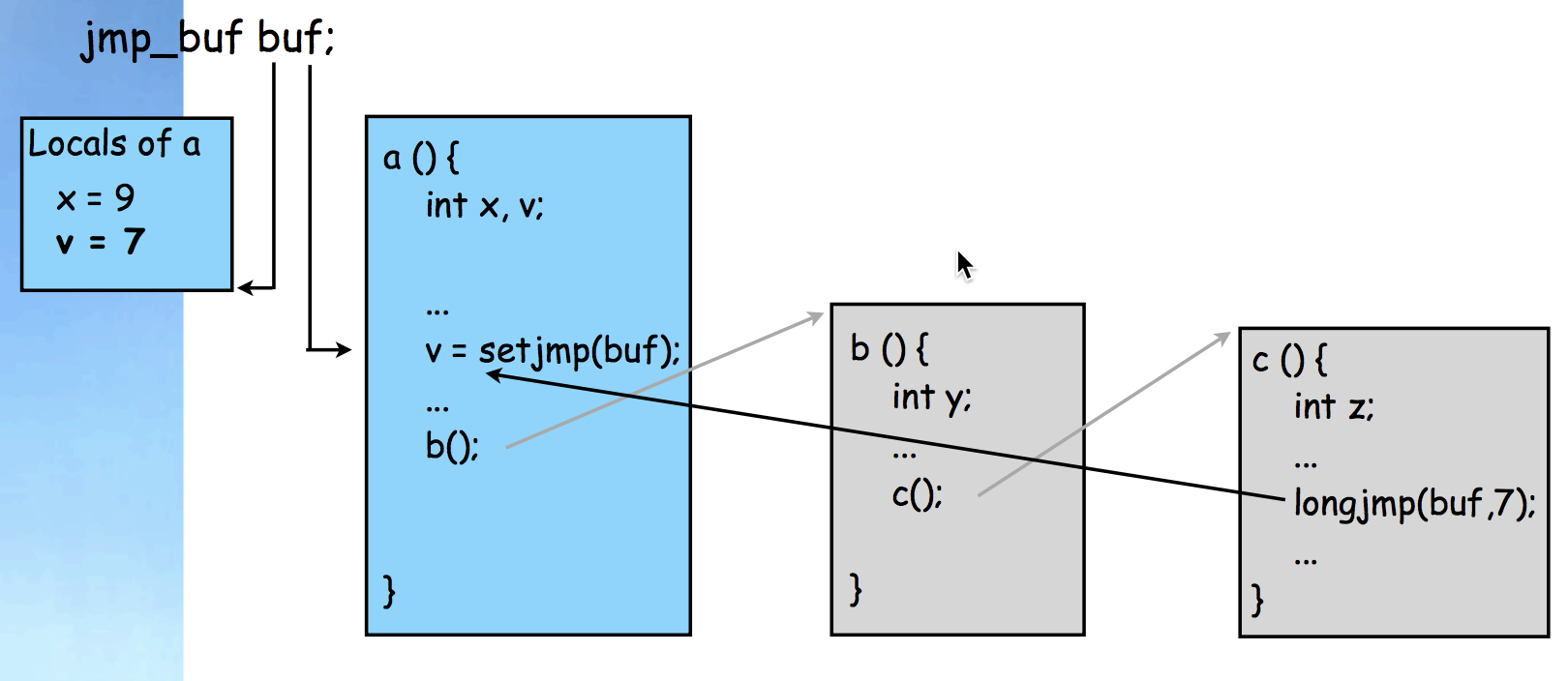setjmp/longjmp 有一个常用的错误用法. 看一个例子程序

```#include <setjmp.h>
#include <stdio.h>
#include <stdlib.h>
int a(char *s, jmp_buf env)
{
int i;
i = setjmp(env);
printf("Setjmp returned -- %d\n", i);
printf("s = %s\n", s);
return i;
}
int b(int i, jmp_buf env)
{
printf("In b: i = %d, Calling longjmp\n", i);
longjmp(env, i);
}
int main()
{
jmp_buf env;
if(a("Bob", env) != 0) exit(0);
b(3,env);
return 0;
}
```

```Setjmp returned -- 0
s = Bob
In b: I = 3, Calling longjmp
Setjmp returned -- 3
s = Bob
```

```Setjmp returned -- 0
s = Bob
In b: i = 3, Calling longjmp
Setjmp returned -- 3
    41956 segmentation fault  ./tmp
```

s 没用正常输出, 为什么呢? 因为 a 函数执行完后, 其栈帧就被销毁掉了, b 函数跳是可以跳回去, 但是 a 中的 局部变量 s 已经被销毁掉了, 再去解引用这块内存, 自然会引起 segme fault 错误. 送上一张清晰明了的图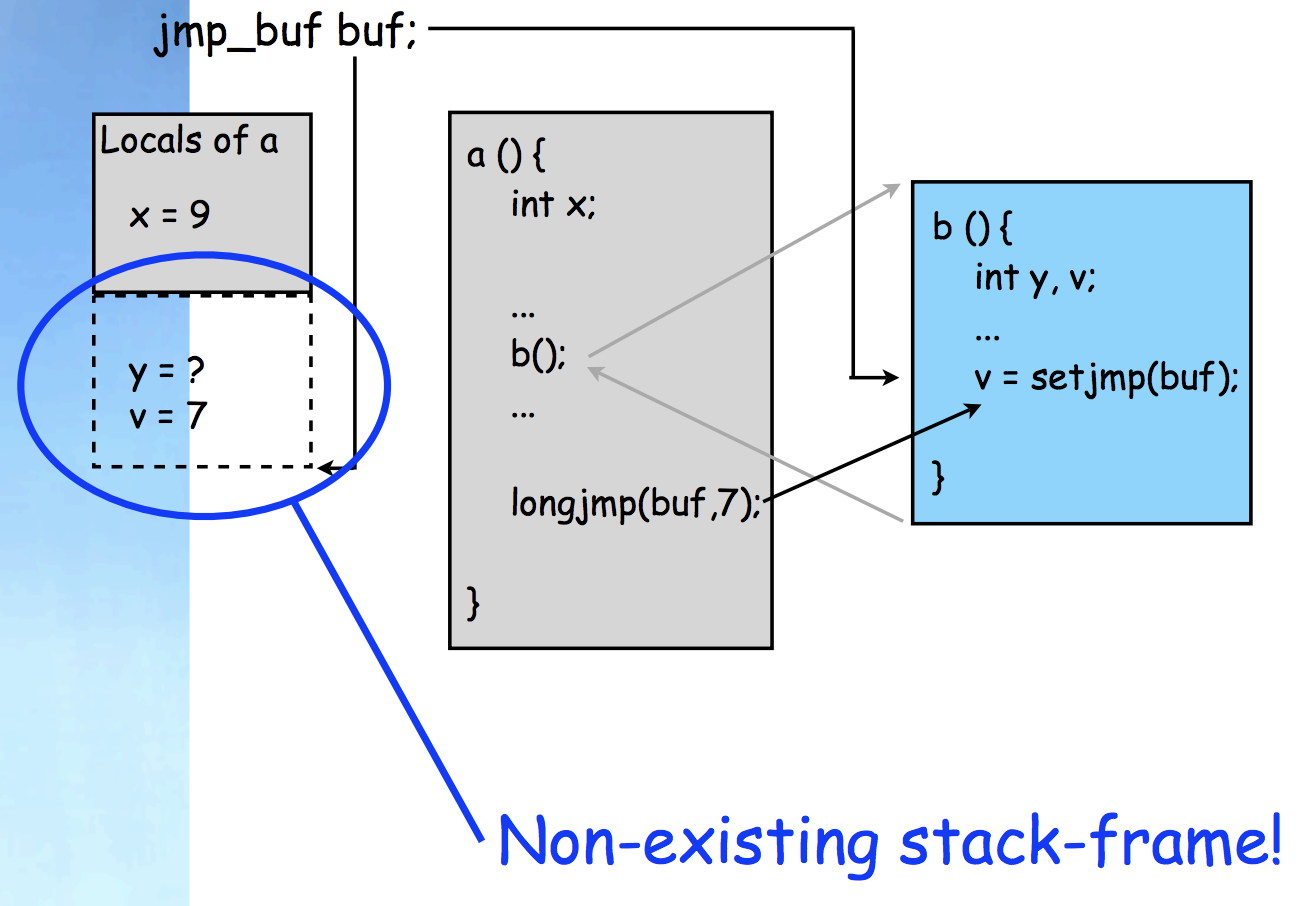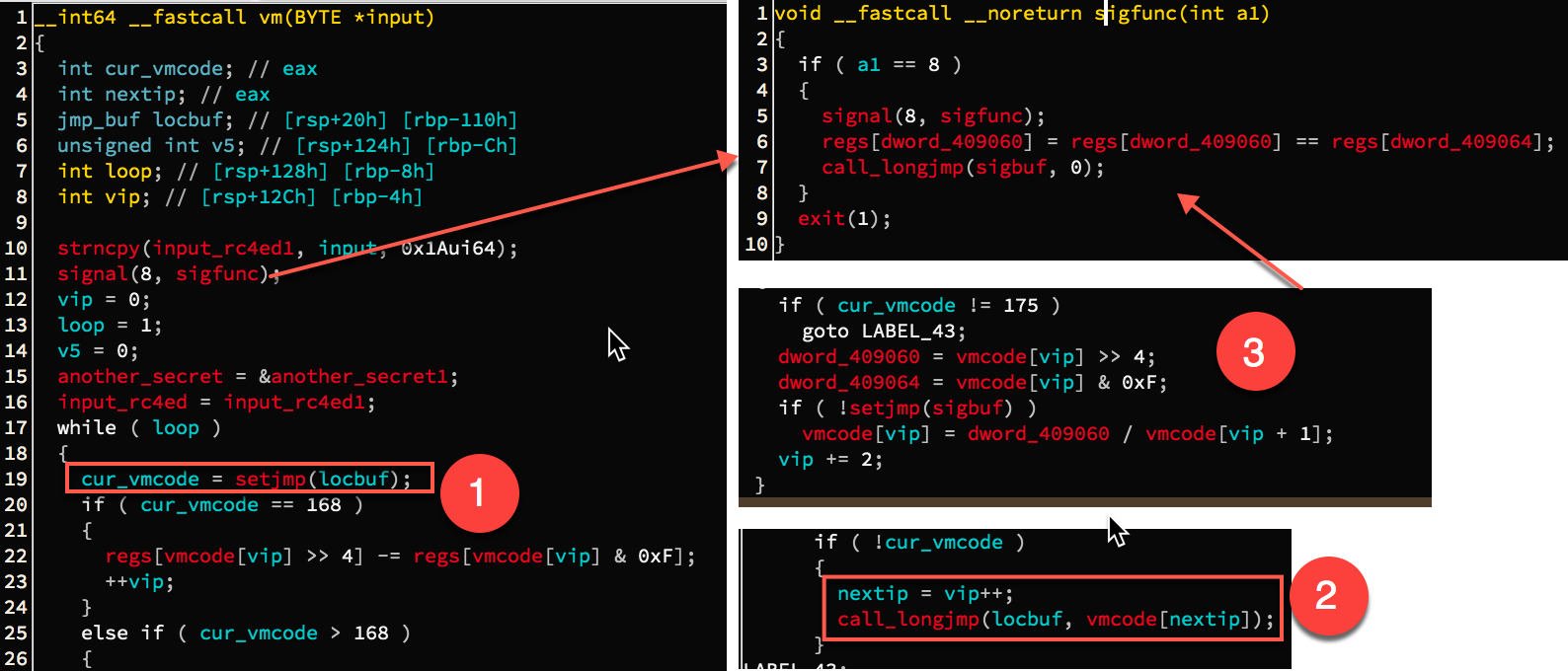```# 0x0000000000402A46 and  0x0000000000402D3C
raxval = idc.get_reg_value('rax')
if(raxval != 0): print '[+] ' + hex(raxval)
```

```[+] 0xabL
[+] 0xabL
[+] 0xabL
[+] 0xaaL
[+] 0xa9L
[+] 0xa0L
[+] 0xabL
[+] 0xa9L
[+] 0xabL
[+] 0xacL
[+] 0xaeL
[+] 0xaaL
[+] 0xaaL
[+] 0xa9L
[+] 0xa0L
[+] 0xafL
402D5E: Integer divide by zero (exc.code c0000094, tid 1016)
[+] 0xa7L
[+] 0xccL
Debugger: thread 1016 has exited (code 0)
```

```0ABh, 3, 0,
0ABh, 4, 1Ah,
0ABh, 0, 66h,
0AAh, 5, 2
0A9h, 53h,
0A0h, 5,
0ABh, 6, 0CCh, 0A9h, 56h, 0ABh,6, 0FFh,
0ACh, 56h,
0AEh, 50h,
0AAh, 6, 5
0AAh, 5, 1,
0A9h, 53h,
0A0h, 5,
0AFh, 56h, 0,
0A7h,1,
0CCh,
0A9h, 35h, 0AAh, 5, 3, 0AFh, 54h, 0, 0A6h
0D1h, 0CCh,0, 0, 0, 0, 0, 0, 0
```

```0ABh, 3, 0,
0ABh, 4, 1Ah,
0ABh, 0, 66h,
0AAh, 5, 2
0A9h, 53h,
0A0h, 5,
0ABh, 6,
0CCh,
0A9h, 56h,
0ABh,6, 0FFh,
0ACh, 56h,
0AEh, 50h,
0AAh, 6, 5
0AAh, 5, 1,
0A9h, 53h,
0A0h, 5,
0AFh, 56h, 0,
0A7h,1,
0CCh,
0A9h, 35h,
0AAh, 5, 3,
0AFh, 54h, 0,
0A6h 0D1h,
0CCh,
0, 0, 0, 0, 0, 0, 0
```

```regs[vmcode[vip]] = vmcode[vip + 1];
vip += 2;
```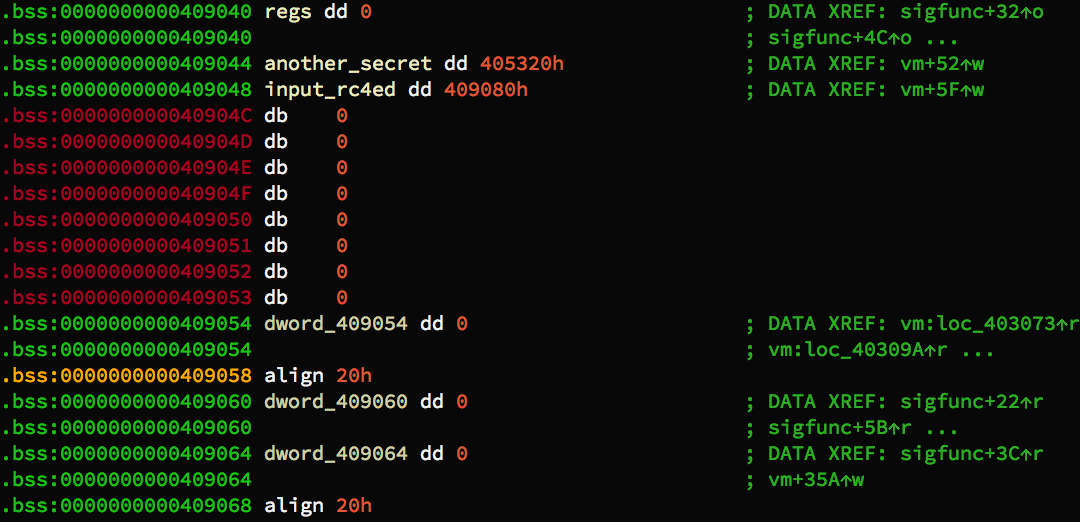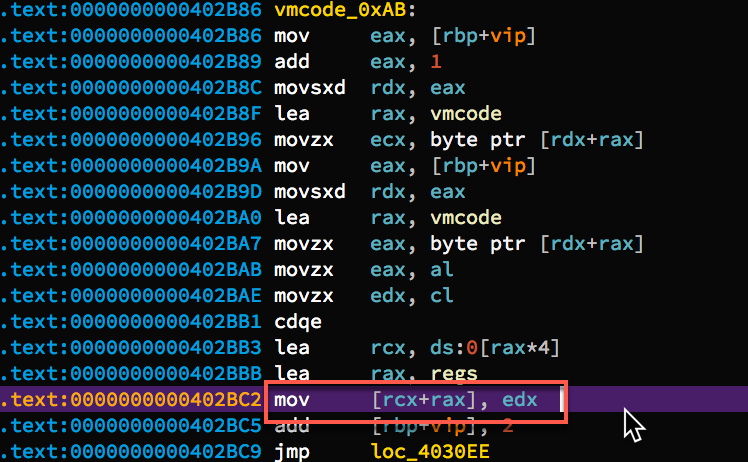```0ABh, 3, 0,     ==> regs = 0
0ABh, 4, 1Ah,   ==> regs = 0x1A
0ABh, 0, 66h,   ==> regs = 0x66

S2:
0AAh, 5, 2      ==> regs = regs
0A9h, 53h       ==> regs[0x53 >>4] += regs[0x53 & 0xF]
0A0h, 5,        ==> regs = regs
0ABh, 6, 0CCh,
0A9h, 56h,
0ABh, 6, 0FFh,
0ACh, 56h,     ==> regs[0x56 >> 4 ] &= regs[0x56 & 0xF]
0AEh, 50h,     ==> regs[0x50 >> 4 ] ^= regs[0x50 & 0xF]
0ADh, 0,       ==> regs = ~LOBYTE(regs)
0AAh, 6,5,
0AAh, 5, 1,
0A9h, 53h,
0A0h, 5,
0AFh, 56h, 0, ==> exception: 第二个操作数作为除数, 产生异常, 进而捕获异常, 执行操作.
==> op1_high = 5 op1_low = 6
==> regs[op1_high] = regs[op1_high] == regs[op1_low];
==> 如果 regs[op1_high] == regs[op1_low], 则 regs[op1_high] = 1

0A7h, 1,      ==> if(regs) {vip += op1; ++vip} else vip += 1
工作流程: vip 指向 0xA7 指令, 虚拟机读入该指令后, vip 自动加 1 指向操作数 1.
执行 0xA7 对应的操作流程时:
1) 如果 regs 为 0, 那么仅仅执行 ++vip, 所以 vip 指向了 0xCC
2) 如果 regs 为 1, 则 vip += op1; ++vip,
这样子的话, 相当于 vip 从当前位置向前移动了 op1 + 1 个位置, 此处为 2,
故跳过了 0xcc 指令, vip 指向了 0xA9. 因而下一条指令执行的是 0xA9.
由于 regs 在上一条指令中由表达式 regs == regs 设置,
所以等价于
if(regs == regs) goto S1;
写成汇编的话就是
cmp regs, regs
jz S1
0CCh,         ==> break out

S1:
0A9h, 35h
0AAh, 5, 3,
0AFh, 54h, 0,
0A6h, 0D1h,  ==> if(!regs) {vip += 0xD1; ++vip} else ++vip;
1) 如果 regs  为 0
这里 0xD1 在汇编指令中对应的是 movsx   eax, al,
将其符号扩展保存到 eax 中, 使用 hex(ctypes.c_int8(0xd1).value) 我们得到 -0x2f.
也就是 vip -= 0x2f, ++vip, 等价于 vip -= 0x2e, 即 vip 前移 0x2e(46) 条指令.
因为现在 vip 指向第一个操作数 0xD1, 所以前移 46 条指令, 正好指向 S2 处.
2) 如果 regs 为 1
此时 ++vip, 于是 vip 指向 0xCC

结合上一条指令, 可以得到
if(regs != regs) goto S2;
写成汇编代码有
cmp regs, regs
jnz S2
0CCh
0 0 0 0 0 0 0
```

```; r1 = another_secret
; r2 = input_rc4ed
mov r3, 0      ;i
mov r4, 0x1A
mov r0, 0x66
S2:
mov r5, r2
mov r5, byte r5 ; input_rc4ed[i]
mov r6, 0xcc
add r5, r6      ; input_rc4ed[i] += 0xcc
mov r6, 0xff    ;
and r5, r6      ; input_rc4ed[i] &= 0xff
xor r5, r0      ; input_rc4ed[i] ^= r0
not r0/m8       ; r0 = ~r0
movzx r0, r0/m8 ;
mov r6, r5      ; chi = input_rc4ed[i]
mov r5, r1      ; #3 chj = another_secret[i]
mov r5, byte r5
cmp r5, r6      ; #2 if(chi == chj) goto S1; else goto STOP;
jz S1
STOP
S1:
add r3, r5      ; r5 is 1 if chi == chj, hence ++r3
mov r5, r3      ; #3 if(i != 0x1A) goto S2; else goto STOP
test r5, r4
jnz S2
STOP
```

```r0 = 0x66
for i in range(0x26):
chi = ((input_rc4ed[i] + 0xcc) & 0xff) ^ r0
r0 = ~ r0
chj = another_secret[i]
if(chi != chj) break
```

```
#! /usr/bin/env python3
#! -*- coding:utf-8 -*-

r0 = 0x66
#0x89, 0xc1, 0xec, 0x50, 0x97, 0x3a, 0x57, 0x59, 0xe4, 0xe6, 0xe4, 0x42, 0xcb, 0xd9, 0x8, 0x22, 0xae, 0x9d, 0x7c, 0x7, 0x80, 0x8f, 0x1b, 0x45, 0x4, 0xe8, 0x0, 0x0, 0x0, 0x0, 0x0, 0x0,
another_secret =[0x89, 0xc1, 0xec, 0x50, 0x97, 0x3a, 0x57, 0x59, 0xe4, 0xe6, 0xe4, 0x42, 0xcb, 0xd9, 0x8, 0x22, 0xae, 0x9d, 0x7c, 0x7, 0x80, 0x8f, 0x1b, 0x45, 0x4, 0xe8, 0x0, 0x0, 0x0, 0x0, 0x0, 0x0]
right_input_rc4d = []
for i in range(0x1A):
for j in range(0xFF + 1):
chi = ((j + 0xCC ) & 0xff) ^ r0
if(chi == another_secret[i]):
right_input_rc4d.append(j)
# Do not write as r0 = ~r0
r0 = (~ r0) & 0xff

print(','.join([hex(i) for i in right_input_rc4d]))
#0x23,0x8c,0xbe,0xfd,0x25,0xd7,0x65,0xf4,0xb6,0xb3,0xb6,0xf,0xe1,0x74,0xa2,0xef,0xfc,0x38,0x4e,0xd2,0x1a,0x4a,0xb1,0x10,0x96,0xa5

```

```238cbefd25d765f4b6b3b6fe174a2effc384ed21a4ab11096a5
```

```
# idapython
# call vm
rc4ed = [0x23,0x8c,0xbe,0xfd,0x25,0xd7,0x65,0xf4,0xb6,0xb3,0xb6,0xf,0xe1,0x74,0xa2,0xef,0xfc,0x38,0x4e,0xd2,0x1a,0x4a,0xb1,0x10,0x96,0xa5]
rcx = idc.GetRegValue('rcx')
i = 0
for addr in range(rcx, rcx + 0x1A):
i = i + 1

```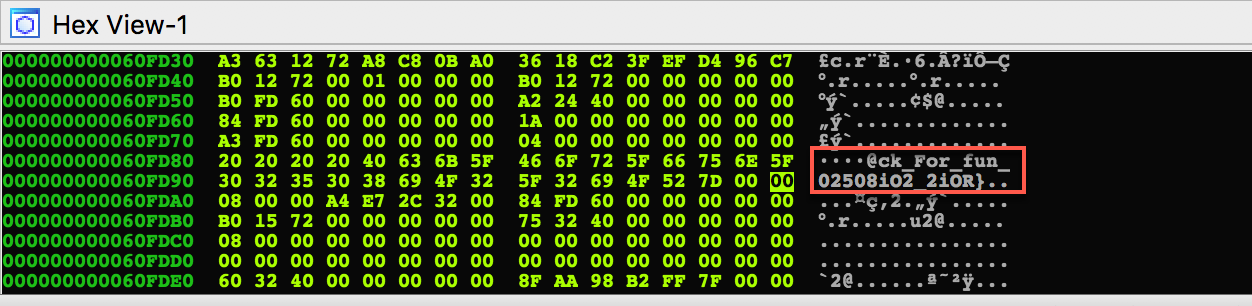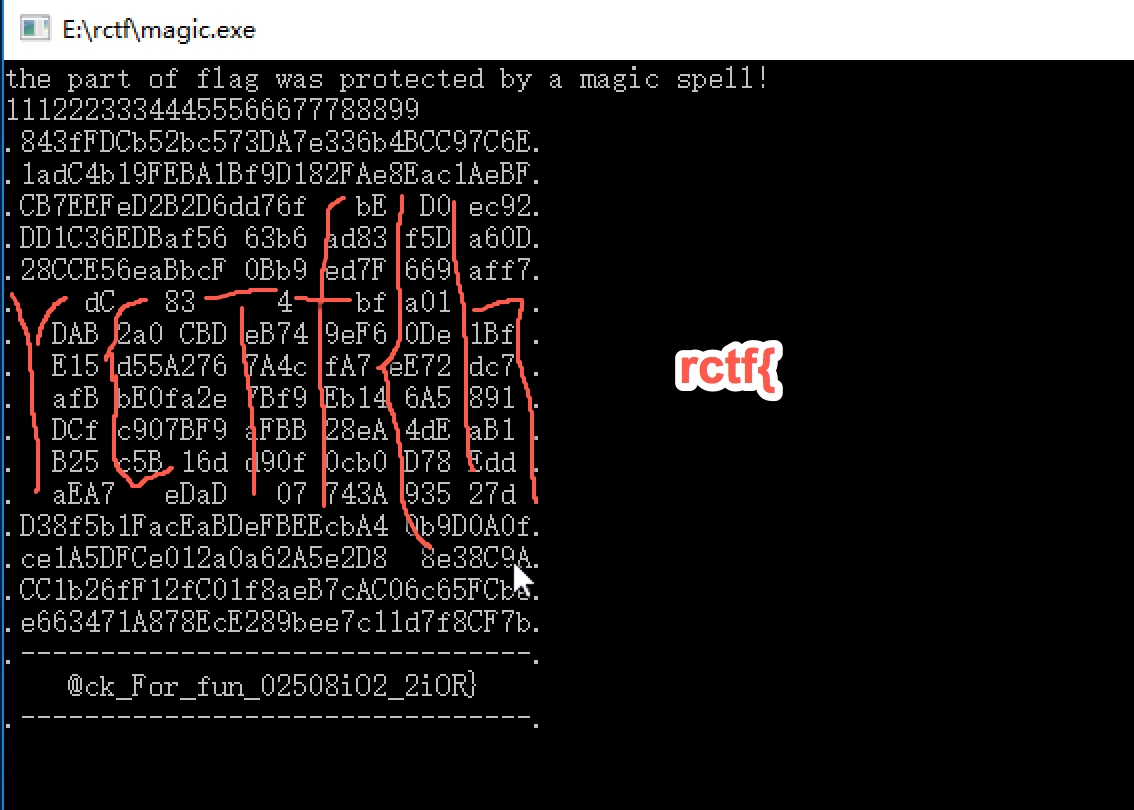# Reference

Contact me by dXAyZ2Vla0AxNjMuY29tCg==
OR